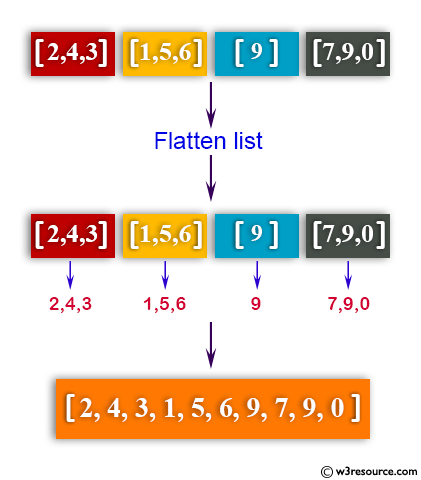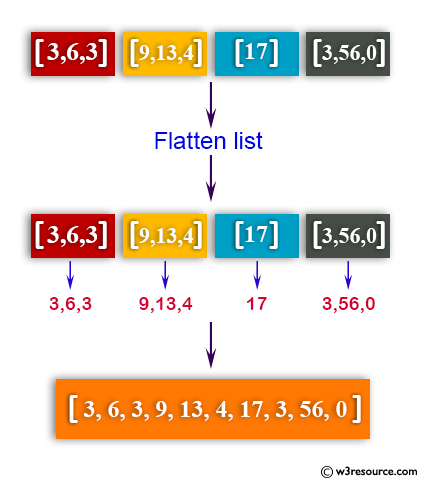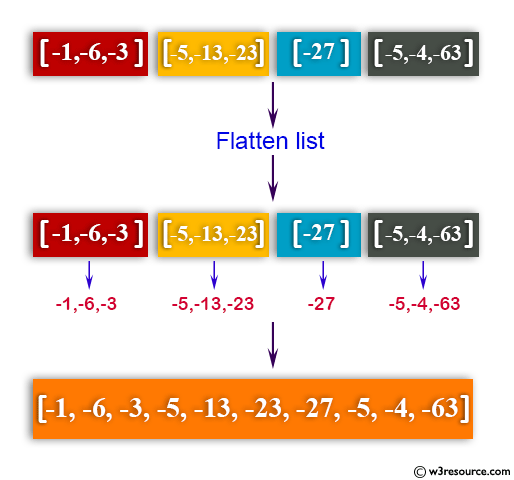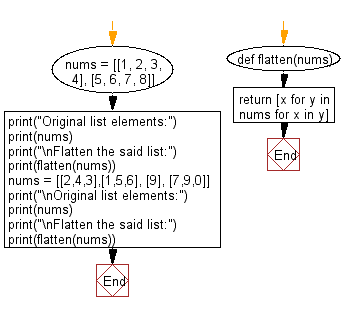﻿ Python: Flatten a shallow list - w3resource# Python: Flatten a shallow list

## Python List: Exercise - 23 with Solution

Write a Python program to flatten a shallow list.

Example - 1 :Example - 2 :Example - 3 :Sample Solution-1:

Python Code:

``````import itertools
original_list = [[2,4,3],[1,5,6], , [7,9,0]]
new_merged_list = list(itertools.chain(*original_list))
print(new_merged_list)
```
```

Sample Output:

```[2, 4, 3, 1, 5, 6, 9, 7, 9, 0]
```

## Visualize Python code execution:

The following tool visualize what the computer is doing step-by-step as it executes the said program:

Sample Solution-2:

Use a list comprehension to extract each value from sub-lists in order.

Python Code:

``````def flatten(nums):
return [x for y in nums for x in y]
nums = [[1, 2, 3, 4], [5, 6, 7, 8]]
print("Original list elements:")
print(nums)
print("\nFlatten the said list:")
print(flatten(nums))
nums = [[2,4,3],[1,5,6], , [7,9,0]]
print("\nOriginal list elements:")
print(nums)
print("\nFlatten the said list:")
print(flatten(nums))
```
```

Sample Output:

```Original list elements:
[[1, 2, 3, 4], [5, 6, 7, 8]]

Flatten the said list:
[1, 2, 3, 4, 5, 6, 7, 8]

Original list elements:
[[2, 4, 3], [1, 5, 6], , [7, 9, 0]]

Flatten the said list:
[2, 4, 3, 1, 5, 6, 9, 7, 9, 0]
```

Flowchart:## Visualize Python code execution:

The following tool visualize what the computer is doing step-by-step as it executes the said program:

Python Code Editor:

Have another way to solve this solution? Contribute your code (and comments) through Disqus.

What is the difficulty level of this exercise?

Test your Python skills with w3resource's quiz

﻿

## Python: Tips of the Day

```print(2_000_000)
```2000000